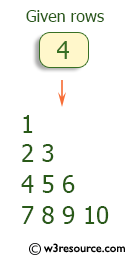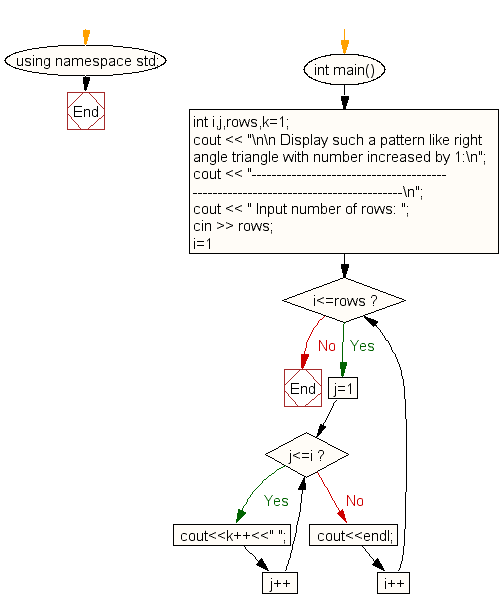﻿ C++ : Right angle triangle pattern with number increase by 1

# C++ Exercises: Display the pattern like right angle triangle with number increased by 1

## C++ For Loop: Exercise-38 with Solution

Write a C++ program to make such a pattern like a right angle triangle with the number increased by 1.

Pictorial Presentation:Sample Solution:

C++ Code :

``````#include <iostream>
#include <string>
using namespace std;

int main()
{
int i,j,rows,k=1;
cout << "\n\n Display such a pattern like right angle triangle with number increased by 1:\n";
cout << "---------------------------------------------------------------------------------\n";
cout << " Input number of rows: ";
cin >> rows;
for(i=1;i<=rows;i++)
{
for(j=1;j<=i;j++)
cout<<k++<<" ";
cout<<endl;
}
}
``````

Sample Output:

``` Display such a pattern like right angle triangle with number increased by 1:
---------------------------------------------------------------------------------
Input number of rows: 4
1
2 3
4 5 6
7 8 9 10
```

Flowchart:C++ Code Editor:

What is the difficulty level of this exercise?

﻿

## C++ Programming: Tips of the Day

Using C++ library in C code

You will need to write an interface layer in C++ that declares functions with extern "C":

```extern "C" int foo(char *bar)
{
returnrealFoo(std::string(bar));
}
```

Then, you will call foo() from your C module, which will pass the call on to the realFoo() function which is implemented in C++.

If you need to expose a full C++ class with data members and methods, then you may need to do more work than this simple function example.

Ref: https://bit.ly/3clHy43

We are closing our Disqus commenting system for some maintenanace issues. You may write to us at reach[at]yahoo[dot]com or visit us at Facebook